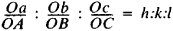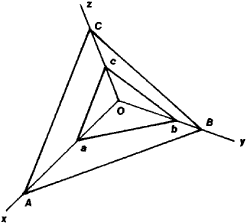# Haüy's Law of Whole Numbers

(redirected from Hauy's Law of Whole Numbers)
The following article is from The Great Soviet Encyclopedia (1979). It might be outdated or ideologically biased.

## Haüy’s Law of Whole Numbers

(also the law of rational intercepts), one of the primary laws of crystallography and also one of the first quantitative laws of the atomic-molecular structure of solids.

Established by R. J. Haüy in 1784, the law asserts that if we take three nonparallel edges of a crystal as the coordinate axes, the position of any face of the crystal can be given in three whole numbers. One of the faces of the crystal abc is arbitrarily chosen as “unity” (see Figure 1); the intercepts Oa, Ob, and Oc, which are cut off by this face at the coordinate edges, are taken as the units of measurement along the coordinate axes. In the general case, the coordinate axes are not at right angles and Oa ≠ Ob ≠ Oc. Acccording to Haüy’s law, for any face of crystal ABC the following relation holds:where OA, OB, and OC are the intercepts formed by face ABC along the coordinate axes (the edges of the crystal) and h, k, and l are three small whole numbers. In crystallography it is accepted practice to describe the edges of a crystal in terms of these numbers (symbols).Figure 1

Haüy’s law of whole numbers establishes a relation between the external shape of a crystal and the principles governing its internal structure, although it was discovered only on the basis of the observation of external shapes of natural crystals long before the main principles of the atomic-molecular theory of the structure of matter were established. The law is a consequence of the fact that the faces of a crystal always correspond to the two-dimensional lattice of a space lattice and the edges of the crystal to the lattice lines. Insofar as the two-dimensional lattices pass through the lattice points, they intercept on the coordinate axes (that is, in the lattice lines) a whole number of lattice periods—that is, the distance between neighboring two-dimensional lattice planes of the three-dimensional lattice. Axial intercepts of the faces also correspond to a whole number of interplanar distances, and therefore the slope of the face is characterized in whole numbers. As a rule, the real faces of a crystal correspond to those two-dimensional lattice planes that have the largest number of atoms per unit area, and therefore these three numbers are not only whole but small as well.

Since the principles governing the periodic distribution of particles in crystals were established, interplanar distances and hence the axial intercepts aA, bB, and cC have been measured from the data obtained by X-ray structural analysis. If the standard selection of coordinate axes developed for crystallography—that is, the natural system of axes, which is determined by the crystal’s symmetry—is used, then the symbols of the faces (hkl), determined on the basis of X-ray structural analysis, coincide to an accuracy of an integral factor with the symbols that are determined on the basis of Haüy’s law of whole numbers according to the slope of the faces of a crystalline polyhedron. The law makes it possible to analytically describe the external shapes of crystals as well as their symmetry and relation to the internal structure.